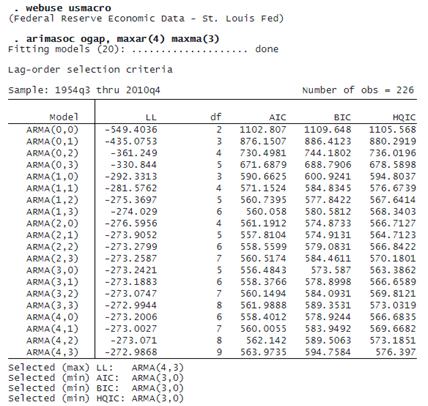MODEL SELECTION FOR ARIMA AND ARFIMA IN ACTION!

We would like to fit an ARMA model for the output gap. We use arimasoc to fit candidate models with a maximum autoregressive lag of 4 and a maximum moving average lag of 3.The output table provides information about each model, including the maximized log likelihood, the number of parameters estimated, and the AIC, BIC, and HQIC.

Below the output table, the selected model from each criterion is listed. The log-likelihood is maximized for the model with the most parameters, the ARMA(4,3). The AIC, BIC, and HQIC all select the more parsimonious ARMA(3,0) model for the output gap. We can now fit our selected model

```. arima ogap, arima(3,0,0)
(output omitted)
```

and proceed to investigate model predictions, forecasts, etc.

Fitting an ARFIMA model instead of an ARIMA model? Instead of typing

```. arimasoc y, maxvar(4) maxma(3)
```

you type

`. arfimasoc y, maxvar(4) maxma(3)`